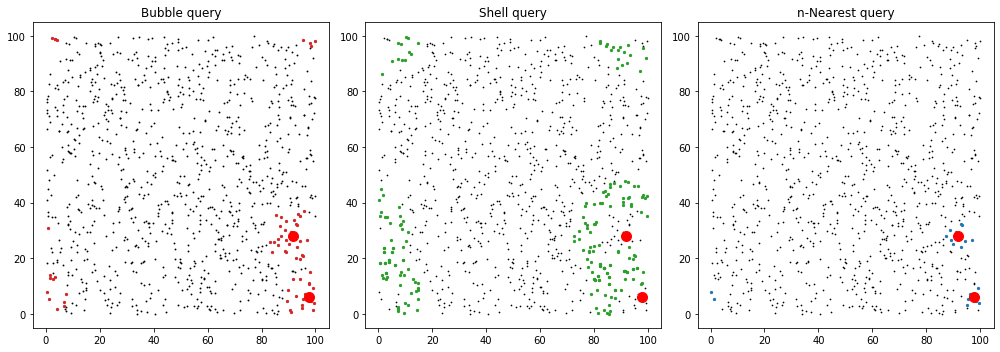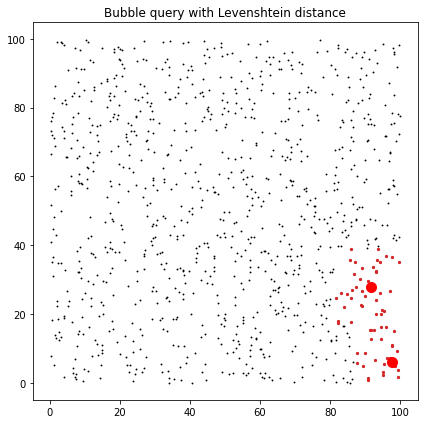# Tutorial¶

## Example with a 2D Uniform Distribution¶

This example generates a 2D random uniform distribution. We will use these points to show how you can use Grid and GriSPy to index and query for neighbors.

Keep in mind that the class Grid provides the funcitonality needed to index the points. The class GriSPy inherits from Grid all its functionalities and adds the relevant methods to perform neighbors queries: set_periodicity, bubble_neighbors, shell_neighbors and nearest_neighbors. For completeness, we will show the example using GriSPy.

 ### Table of Contents * Create a random distribution of points * Index the points with Grid/GriSPy * Creating your curstom distance function

# Create a random distribution of points¶

## Import GriSPy and others packages¶

:

import numpy as np
import matplotlib.pyplot as plt

import grispy as gsp


## Create random points and centres¶

:

Npoints = 10 ** 3
Ncentres = 2
dim = 2
Lbox = 100.0

rng = np.random.default_rng(seed=0)
data = rng.uniform(0, Lbox, size=(Npoints, dim))
centres = rng.uniform(0, Lbox, size=(Ncentres, dim))


# Index the points with Grid/GriSPy¶

:

grid = gsp.GriSPy(data, N_cells=32)


You can get some information about the grid using some helpfull methods:

:

grid.shape   # number of cells per dimension

:

(32, 32)

:

grid.edges   # grid edges in each dimension

:

array([[2.97613690e-01, 1.90001607e-02],
[9.99501352e+01, 9.98067457e+01]])

:

digits = grid.cell_digits(centres)   # check the cell indices (or digits) where a given set of points would fall
digits

:

array([[31,  1],
[29,  8]], dtype=int16)


This means that from the 2 input points, the first would be located in the cell (31, 1) and the second in (29, 8).

:

grid.cell_count(digits)   # number of points in the array data that where indexed at initialization

:

array([2, 0])

:

grid.cell_centre(digits)    # position of each cell centre

:

array([[98.39306458,  4.69655073],
[92.16478198, 26.52512006]])


The digits of cells can be used in other useful methods like cell_walls or cell_points.

Another important method is contains which shows if a given set of new points are contained by the grid.

:

points = np.array([
[30., 50.],
[-10., -10.]
])
grid.contains(points)

:

array([ True, False])


## Set periodicity conditions¶

Set periodicity conditions on x-axis (or axis=0) and y-axis (or axis=1)

:

periodic = {0: (0, Lbox), 1: (0, Lbox)}
grid.set_periodicity(periodic, inplace=True)
grid

:

GriSPy(N_cells=32, copy_data=False, periodic={0: (0, 100.0), 1: (0, 100.0)}, metric='euclid')


Also you can build a periodic grid in the same step

:

grid = gsp.GriSPy(data, periodic=periodic)


Important: Periodic boundaries don’t have to be exactly the same as the values of grid.edges. The edges of the grid are computed from real data to optimize the queries on sparse data. So, there is nothing wrong on the edge of the grid being at 99.9 but the periodic boundary set exactly at 100.

## Query for neighbors within upper_radii¶

:

upper_radii = 10.0
bubble_dist, bubble_ind = grid.bubble_neighbors(
)


:

upper_radii = 20.0
shell_dist, shell_ind = grid.shell_neighbors(
centres,
)


## Query for nth nearest neighbors¶

:

n_nearest = 10
near_dist, near_ind = grid.nearest_neighbors(centres, n=n_nearest)


## Plot results¶

:

fig, axes = plt.subplots(1, 3, figsize=(14, 5))

ax = axes
ax.set_title("Bubble query")
ax.scatter(data[:, 0], data[:, 1], c="k", marker=".", s=3)
for ind in bubble_ind:
ax.scatter(data[ind, 0], data[ind, 1], c="C3", marker="o", s=5)
ax.plot(centres[:,0],centres[:,1],'ro',ms=10)

ax = axes
ax.set_title("Shell query")
ax.scatter(data[:, 0], data[:, 1], c="k", marker=".", s=2)
for ind in shell_ind:
ax.scatter(data[ind, 0], data[ind, 1], c="C2", marker="o", s=5)
ax.plot(centres[:,0],centres[:,1],'ro',ms=10)

ax = axes
ax.set_title("n-Nearest query")
ax.scatter(data[:, 0], data[:, 1], c="k", marker=".", s=2)
for ind in near_ind:
ax.scatter(data[ind, 0], data[ind, 1], c="C0", marker="o", s=5)
ax.plot(centres[:,0],centres[:,1],'ro',ms=10)

fig.tight_layout()# Creating your curstom distance function¶

Let’s assume that we intend to compare our distances using levenshtein’s metric for similarity between text (https://en.wikipedia.org/wiki/Levenshtein_distance).

Luckly we have the excellent textdistance library that implements efficiently this distance.

We can install it with

\$ pip install textdistance


and then import it with

:

import textdistance


So to make these custom distance compatible with GriSPy, we must define a function that receives 3 parameters:      - c0 the center to which we seek the distance. - centres the $$C$$ centers to which we want to calculate the distance from    a c0. - dim the dimension of each center and c0.

Finally the function must return a np.ndarray with $$C$$ elements where the element $$j-nth$$ corresponds to the distance between c0 and centres$$_j$$.

:

def levenshtein(c0, centres, dim):
# textdistance only operates over list and tuples
c0 = tuple(c0)

# creates a empty array with the required
# number of distances
distances = np.empty(len(centres))
for idx, c1 in enumerate(centres):

# textdistance only operates over list and tuples
c1 = tuple(c1)

# calculate the distance
dis = textdistance.levenshtein(c0, c1)

# store the distance
distances[idx] = dis

return distances


Then we create the grid with the custom distance, and run the code

:

grid = gsp.GriSPy(data, metric=levenshtein)

lev_dist, lev_ind = grid.bubble_neighbors(


Finally we can check our bubble_neighbors result with a plot

:

fig, axes = plt.subplots(figsize=(6, 6))

ax = axes
ax.set_title("Bubble query with Levenshtein distance")
ax.scatter(data[:, 0], data[:, 1], c="k", marker=".", s=3)
for ind in lev_ind:
ax.scatter(data[ind, 0], data[ind, 1], c="C3", marker="o", s=5)
ax.plot(centres[:,0],centres[:,1],'ro',ms=10)

fig.tight_layout()